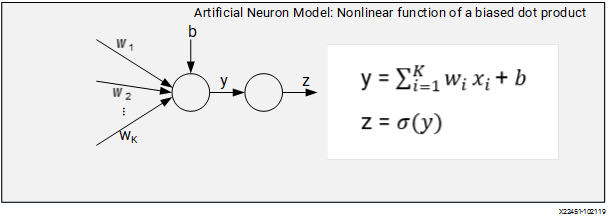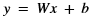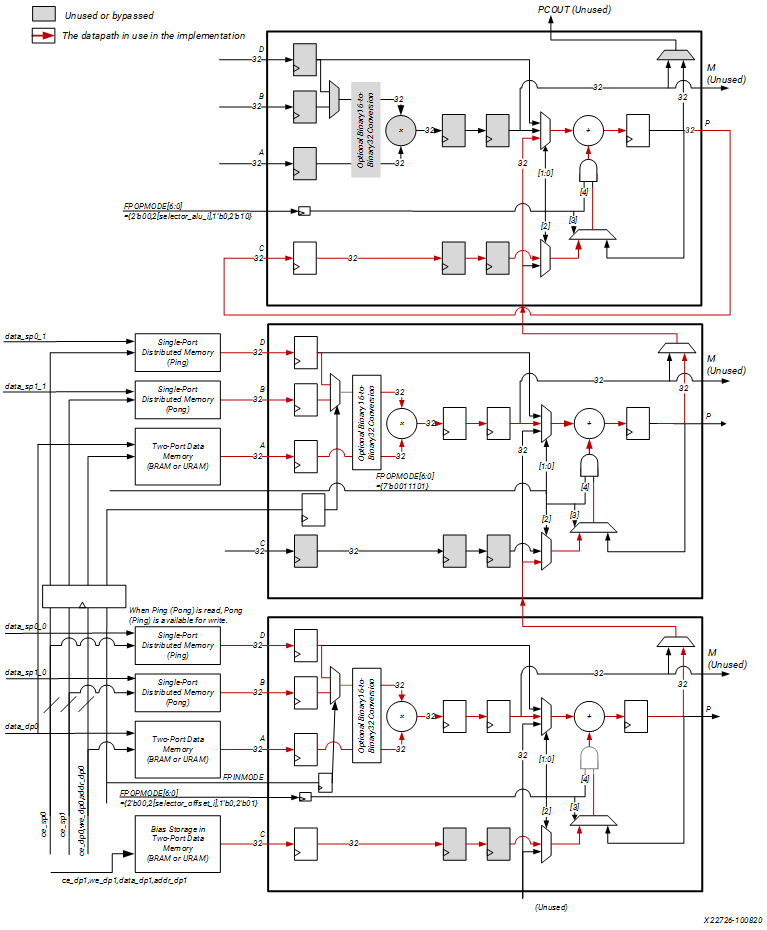# Floating Point Time-Interleaved Dot-Product Engine

## Versal ACAP DSP Engine Architecture Manual (AM004)

Document ID
AM004
Release Date
2022-09-11
Revision
1.2.1 English

A neuron model can be represented as a nonlinear function of a biased dot product of a weight vector. A simple representation is shown in the following figure.

Figure 1. Artificial Neuron Broadcast ModelMultiple artificial neurons are modeled as a matrix-vector multiplication. The weights are in an M x K matrix W, the biases are in an M-dimensional vector b, and the input data (activations) are in an K-dimensional vector x. The pre-activations are therefore in an M dimensional vector represented as follows.The following figure implements the biased vector dot products with a cascade of DSPs. For N=64, in the bottom two DSPs, each computes a dot product for two 32-D input vectors and the bottom DSP also adds the bias term. The top DSP accumulates the results using a two threads accumulator loop between P and C.

Figure 2. Floating-Point Time-Interleaved Dot-Product EngineNote the following in the use case design.

• The input and bias vectors are implemented as dual-port memories (Block RAM) where as the weight vectors are single port memories implemented in programmable logic using ping-pong scheme.
• The block diagram shows one column of cascading DSPs. In the design, there are two columns that share the input vectors in the same cycle in both the middle and bottom rows of DSPs.
• There is a separate memory for bias in each column. The memories share the same controls (CE, WE, and Addr) but separate data inputs.
• The control signals to data/weight memories and FPINMODE to the middle DSPs are delayed (registered) versions of the ones to the bottom DSPs except the input data which are dedicated to each memory.

The reference design files associated with this use case are available in the fp_time_int_dot_product directory in the associated design archive file, am004-versal-dsp-engine.zip.